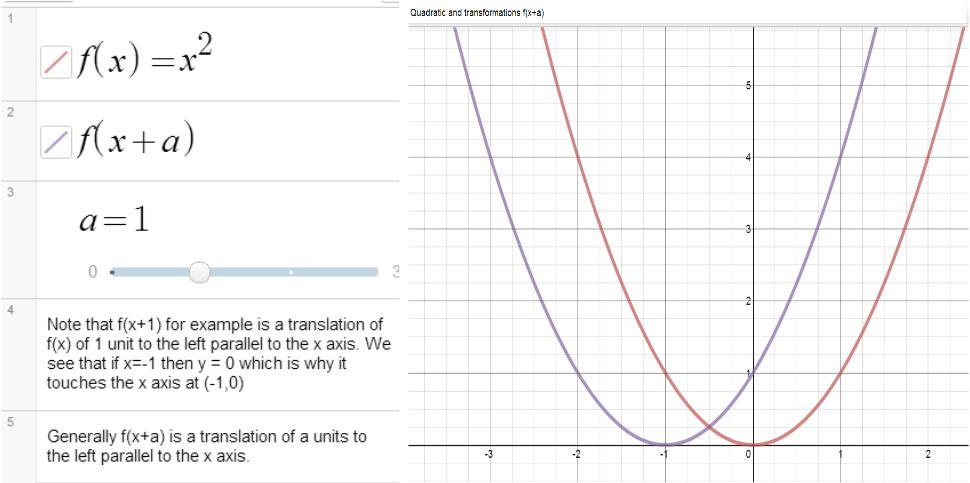# Transformations

A summary and pages to experiement with:Note how easy it is using the Desmos graphing calculator to show a graph and then the same graph after a transformation. For example see here the graph of x2 and (x+a)(or click on  the image).

As you can see all you need do is define f(x) which then gives you the ability to graph transformations of f(x). Using a slider means students can explore what happens if the value of a changes. In this particular example a is restricted to integer values from 0 to 3 but this is very easy to change by editing the slider; simply click on the numbers at the ends of the slider and choose the values required, you can also choose the step size.

I like the ability to add text to graph pages which means it is possible to add a few words of explanation or even questions for students.

I have used these prompts before in a computer room, the aim being for students to explore and generalize. The headings are links to Desmos graph pages students could experiment with.

Transformations Exercise

For linear, quadratic and the sine and cosine functions the following transformations should be understood:

y=f(x)+a trigonometric
Eg plot y = x2   and y = x+ 3   on the same diagram, compare the graphs.
Plot y = x− 4, y = x− 1
Compare y = x2  and y = x+ k where k is any integer, positive or negative.
Also compare y = sin x and y = sin x + 3.
Compare y = sin x and y = sin x + k where k is any number

2. y = f(x+a) quadratic

y = f(x+a) trigonometric
Eg plot  y = x2   and y = (x+ 3)on the same diagram, compare the graphs.
Compare y = x2   and y = (x−3)2.  Compare y = x2   and y = (x−4)2
Compare y = x2  and y =(x+ k)2 where k is any integer, positive or negative.
Compare y = sin x and y = sin (x+90) , sin (x – 90), sin(x+180)
Compare y = cos x with y = cos(x+90), cos (x-90)

3. y = af(x) trigonometric
Compare y = sinx and y = 2sinx.
Compare y = cosx and y = 3cosx and y =  0.5cos x
Compare y=x2 and kx2 where k is any integer positive or negative.

4. y = f(ax) trigonometric
Compare y = sinx and y = sin2x. Compare y = sin x and y = sin(0.5x).
Compare y = cos x and y = cos 3x. Compare y = cos x and y = cos(0.5x)